Note: This is a working paper which will be expanded/updated frequently. The directory deleeuwpdx.net/pubfolders/secstress has a pdf copy of this article, the complete Rmd file that includes all code chunks, and R files with the code. Suggestions are welcome 24/7.

# 1 Problem

Define the multidimensional scaling (MDS) loss function $\begin{equation} \sigma_r(x)=\sum_{i=1}^nw_i(\delta_i-(x'A_ix)^r)^2,\label{E:rstress} \end{equation}$

with $$r>0$$ and the $$A_i$$ positive semi-definite. The $$w_i$$ are positive weights, the $$\delta_i$$ are non-negative dissimilarities. We call this rStress (De Leeuw, Groenen, and Mair (2016)). Special cases are stress (Kruskal 1964) for $$r=\frac12$$, sstress (Takane, Young, and De Leeuw 1977) for $$r=1$$, and the loss function used in MULTISCALE (Ramsay 1977) for $$r\rightarrow 0$$.

In this paper we are interested in the first and second derivatives of rStress, and in the various applications of these derivatives to the problem of minimizing rStress.

# 2 Derivatives

Compact expression for the first and second derivatives of $$\sigma_r$$ can be given by defining the matrices \begin{align} B_r(x)&:=\sum_{i=1}^n w_i\delta_i(x'A_ix)^{r-1}A_i,\label{E:bdef}\\ C_r(x)&:=\sum_{i=1}^n w_i(x'A_ix)^{2r-1}A_i,\label{E:cdef}\\ S_r(x)&:=\sum_{i=1}^nw_i\delta_i(x'A_ix)^{r-1}\left[A_i+2(r-1)\frac{A_ixx'A_i}{x'A_ix}\right],\label{E:sdef}\\ T_r(x)&:=\sum_{i=1}^nw_i(x'A_ix)^{2r-1}\left[A_i+2(2r-1)\frac{A_ixx'A_i}{x'A_ix}\right].\label{E:tdef} \end{align} We then have $\begin{equation} \mathcal{D}\sigma_r(x)=-4r\{B_r(x)-C_r(x)\}x,\label{E:grad} \end{equation}$ and $\begin{equation} \mathcal{D}^2\sigma_r(x)=-4r\{S_r(x)-T_r(x)\}.\label{E:hess} \end{equation}$

Note that $$S_r(x)$$ is positive semi-definite for $$r\geq\frac12$$ and $$T_r(x)$$ is positive-semi-definite for $$r\geq\frac14$$.

We have written the R function mdsDerivatives() to evaluate the gradient and Hessian. Just to make sure our formulas are correct, the code can optionally compute numerical derivatives using the numDeriv package of Gilbert and Varadhan (2014).

# 3 Newton’s Method

Newton’s method to minimize rStress is $\begin{equation} x^{(k+1)}=x^{(k)}-[S_r(x^{(k)})-T_r(x^{(k)})]^{-1}(B_r(x^{(k)})-C_r(x^{(k)}))x^{(k)}. \end{equation}$

As we can expect in highly nonlinear situations like MDS, Newton’s method without safeguards sometimes works and sometimes doesn’t. If it works, it is generally fast, which is of some interest at least because the majorization method developed in De Leeuw, Groenen, and Mair (2016) for minimizing rStress can be very slow, especially for $$r\geq\frac12$$.

# 4 Dutch Political Parties

Our main example in the paper is are the dissimilarity measures for nine Dutch political parties, collected by De Gruijter (1967).

##       KVP PvdA  VVD  ARP  CHU  CPN  PSP   BP
## PvdA 5.63
## VVD  5.27 6.72
## ARP  4.60 5.64 5.46
## CHU  4.80 6.22 4.97 3.20
## CPN  7.54 5.12 8.13 7.84 7.80
## PSP  6.73 4.59 7.55 6.73 7.08 4.08
## BP   7.18 7.22 6.90 7.28 6.96 6.34 6.88
## D66  6.17 5.47 4.67 6.13 6.04 7.42 6.36 7.36
Newton’s method converges in all cases, although it often behaves very erratically in the early iterations. Table 1 shows the number of iterations, the rStress value, the maximum norm of the gradient, and the smallest eigenvalue of the Hessian at the solution.
## r:  0.40  iters:     60  rStress:  0.07488639  maxGrad:  0.00000000  minHess:  -23.37023839
## r:  0.45  iters:     13  rStress:  0.06911461  maxGrad:  0.00000000  minHess:  -7.68219018
## r:  0.50  iters:     48  rStress:  0.15944252  maxGrad:  0.00000003  minHess:  -12.72852418
## r:  0.55  iters:     77  rStress:  0.12871421  maxGrad:  0.00000000  minHess:  -6.61452301
## r:  0.65  iters:     55  rStress:  0.07731578  maxGrad:  0.00000000  minHess:  -0.00000000
## r:  0.75  iters:      9  rStress:  0.14507211  maxGrad:  0.00000000  minHess:  -3.12532160
## r:  0.90  iters:     60  rStress:  0.13989729  maxGrad:  0.00000003  minHess:  -0.00000000
## r:  1.00  iters:     26  rStress:  0.14925820  maxGrad:  0.00000000  minHess:  -0.00000000
## r:  2.00  iters:     47  rStress:  0.35796584  maxGrad:  0.00000000  minHess:  -0.00000000
Table 1: Newton solutions with various r

Clearly for the majority of solutions Newton stops at a saddle point, or at least a flat spot fairly close to a local minimum. Only for large values of r do we find a proper local minimum. For values of r less than .40 we cannot get Newton to work. It rapidly diverges into regions with very large values of both $$x$$ and rStress. The configurations in figure 1 also seem to differ quite a bit for smaller values of r. Note the increased clustering for increasing r, until finally for $$r=2$$ parties are put in the edges of an equilateral triangle.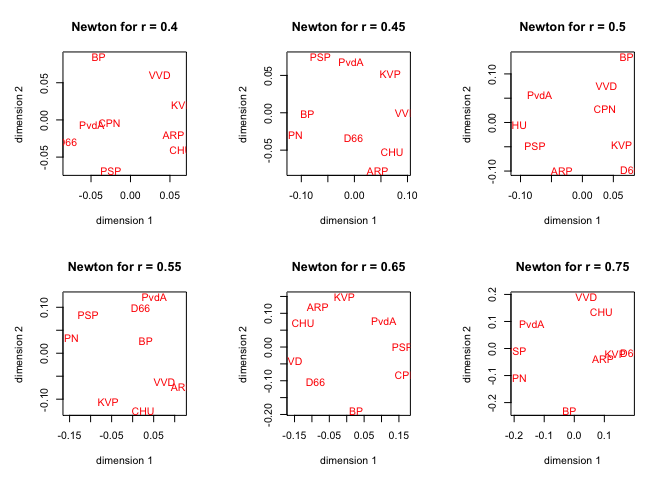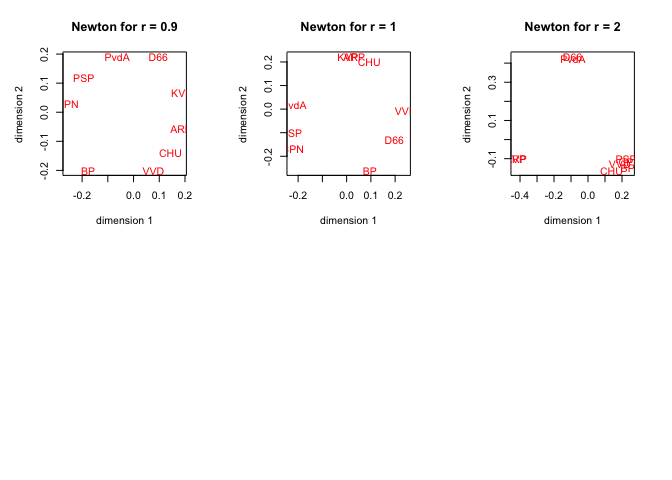Figure 1: Newton configurations with various r

# 5 Majorizing Newton

In this section we limit ourselves to the case $$r\geq\frac12$$. Without loss of generality we assume the dissimilarities are scaled by $\begin{equation} \sum_{i=1}^nw_i\delta_i^2=1. \end{equation}$ Next, it is convenient to define $\begin{equation} \rho_r(x):=\sum_{i=1}^nw_i\delta_i(x'A_ix)^r, \end{equation}$ and $\begin{equation} \eta_r(x):=\sum_{i=1}^nw_i(x'A_ix)^{2r}, \end{equation}$ so that $\begin{equation} \sigma_r(x)=1-2\rho_r(x)+\eta_r(x).\label{E:expand} \end{equation}$ If $$r\geq\frac12$$ then both $$\rho_r$$ and $$\eta_r$$ are convex (De Leeuw, Groenen, and Mair (2016)). Thus $\begin{equation} \rho_r(x)\geq\rho_r(y)+(x-y)'\mathcal{D}\rho(y), \end{equation}$ for all $$x$$ and $$y$$, which translates to the majorization $\begin{equation} \sigma_r(x)=\min_y \zeta(x,y), \end{equation}$ where $\begin{equation} \zeta(x,y):=1-2\rho_r(y)-4r(x-y)'B_r(y)y +\eta_r(x). \end{equation}$ Now consider the algorithm where we use block relaxation to alternate over minimization over $$\zeta$$ over $$x$$ and $$y$$. By definition $\begin{equation} \text{arg}\min_y\zeta(x,y)=x, \end{equation}$

so minimization over $$y$$ for given $$x$$ is trivial. We minimize $$\zeta$$ over $$x$$ for given $$y$$ by using one or more steps of Newton’s method, relying on the fact that $$\zeta$$ is convex in $$x$$ for given $$y$$. Thus there will be no local minima problem with Newton, although we may observe non-convergence. Note that it will not be neceesary for convergence to iterate Newton to convergence between updates of $$y$$. In fact we propose an algorithm in which only a single Newton step is done.

The derivatives needed for the Newton steps are $\begin{equation} \mathcal{D}_1\zeta(x,y)=-4r(B_r(y)y-C_r(x)x), \end{equation}$ and $\begin{equation} \mathcal{D}_{11}\zeta(x,y)=4rT_r(x). \end{equation}$ Thus the two-block algorithm with a single Newton step becomes \begin{align} y^{(k)}&=x^{(k)},\\ x^{(k+1)}&=x^{(k)}-[T_r(x^{(k)})]^{-1}(B_r(y^{(k)})y^{(k)}-C_r(x^{(k)})x^{(k)}), \end{align} but this is of course equivalent to the algorithm $\begin{equation} x^{(k+1)}=x^{(k)}-[T_r(x^{(k)})]^{-1}(B_r(x^{(k)})-C_r(x^{(k)}))x^{(k)}. \end{equation}$

This is what we have implemented in our R program, using the parameter linearize=TRUE. By default linearize=FALSE, which is the standard uncorrected Newton method.

The idea is to give up some speed (and quadratic convergence) by gaining stability. In table 2 we do see larger numbers of iterations (but iterations are marginally faster because they do not need $$S_r(x)$$). We also have observed monotone convergence of loss function values in all cases, and we see that convergence is always to a local minimum. In most cases, except for $$r=1$$, the solution found has a lower loss function value than the one found by the Newton method. Remember, however, that our majorization method is only guaranteed to work for $$r\geq\frac12$$.

## r:  0.40  iters:    288  rStress:  0.02854517  maxGrad:  0.00000011  minHess:  -0.00000000
## r:  0.45  iters:    268  rStress:  0.03823655  maxGrad:  0.00000009  minHess:  -0.00000000
## r:  0.50  iters:    729  rStress:  0.04460338  maxGrad:  0.00000011  minHess:  -0.00000000
## r:  0.55  iters:    186  rStress:  0.05524495  maxGrad:  0.00000009  minHess:  -0.00000000
## r:  0.65  iters:    104  rStress:  0.07731578  maxGrad:  0.00000006  minHess:  -0.00000000
## r:  0.75  iters:     96  rStress:  0.10711307  maxGrad:  0.00000006  minHess:  -0.00000000
## r:  0.90  iters:    150  rStress:  0.13989729  maxGrad:  0.00000005  minHess:  -0.00000000
## r:  1.00  iters:   1000  rStress:  0.15444014  maxGrad:  0.00000008  minHess:  -0.00000000
## r:  2.00  iters:     53  rStress:  0.23176557  maxGrad:  0.00000005  minHess:  -0.00000000
Table 2: Majorization solutions with various r

The configurations found by the majorization method are more stable over different values of r, and show the familar effect of becoming more and more clustered if r increases. Note that for $$r=2$$ the majorization method finds a better location of the parties to the edges, although finding the optimum allocation is of course a combinatorial problem.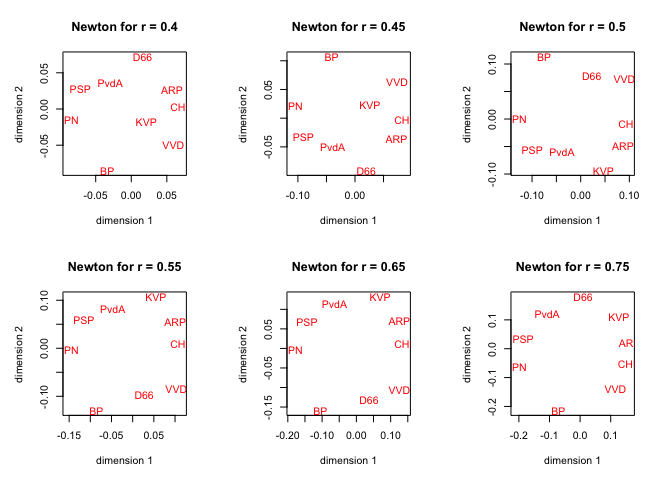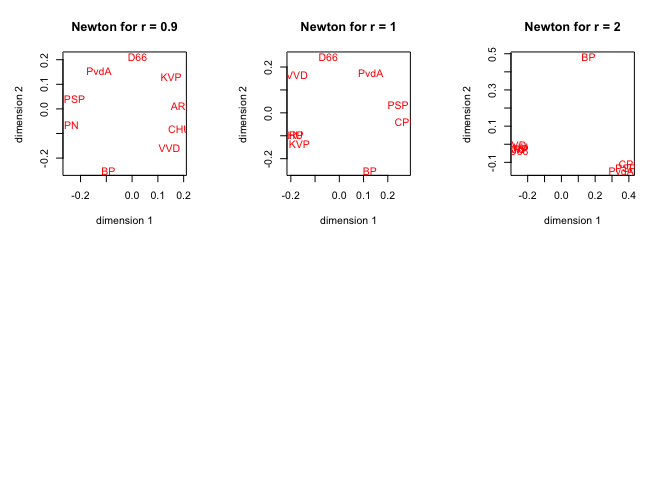Figure 2: Majorization with various r

In table 3 we give the rStress values and iteration numbers for the scalar majorization algorithm of De Leeuw, Groenen, and Mair (2016). We see that the rStress values for $$r\geq\frac12$$ are basically the same as the ones from the majorization algorithm in this paper, but the number of iterations is much larger. In fact, it is larger than 100,000 for $$r=1$$ and $$r=2$$.
## r:  0.10  iters:  29103  rStress:  0.00546400
## r:  0.25  iters:   3605  rStress:  0.00631000
## r:  0.50  iters:   3566  rStress:  0.04460300
## r:  0.75  iters:   3440  rStress:  0.10711300
## r:  1.00  iters:     NA  rStress:  0.15539200
## r:  2.00  iters:     NA  rStress:  0.23487700
Table 3: Scalar majorization solutions with various r

# 6 Sensitivity Analysis

The second derivatives can also be used to draw sensitivity regions around points in an MDS solution. At a point $$x$$ where the first derivatives vanish and the Hessian is positive semi-definite, we have $\begin{equation} \sigma_r(y)\approx\sigma_r(x)+\frac12 (x-y)'\mathcal{D}^2\sigma_r(x)(x-y), \end{equation}$ and thus $$\{y\mid\sigma_r(y)\leq\alpha\}$$ is approximately the ellipsoid $\begin{equation} \{y\mid(x-y)'\mathcal{D}^2\sigma_r(x)(x-y)\leq 2(\alpha-\sigma_r(x))\}. \end{equation}$

For graphics in the plane we take $$2\times 2$$ principal submatrices of the Hessian and draw ellipses, for example by using the R package car (Fox and Weisberg (2011)). We have to remember that in car the shape matrix is the inverse of our second derivative matrix, while their radius parameter corresponds with our $$\sqrt{2(\alpha-\sigma_r(x))}$$.

We illustrate this with the majorization solution for $$r=\frac12$$, which has rStress 0.0446034. In figure 3 we choose $$\alpha-\sigma_r=.001$$, which means we look for the solutions which have rStress larger than 0.0446034 by 0.001 or less.

## Loading required package: carData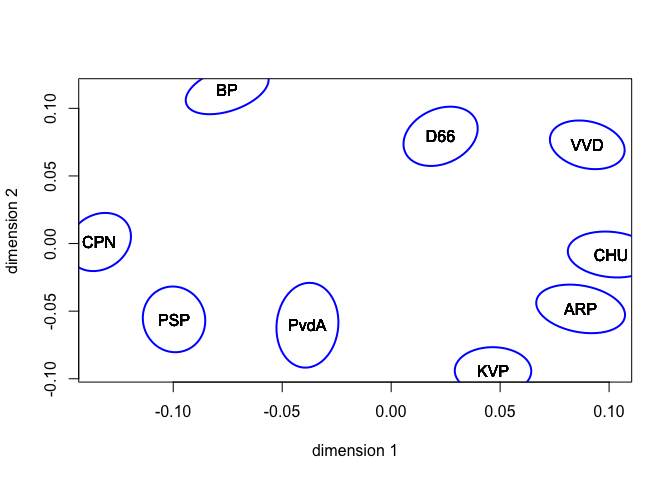Figure 3: Sensitivity regions for r = 0.5

# 7 Nonmetric MDS

Our main function newtonMe() has parameter nonmetric, by default FALSE, and ties, by default "primary". It uses the algorithm from De Leeuw (2016) to perform a monotonic regression after updating the configuration. We start with three runs for $$r=\frac12$$. The first is Newton, the second Newton with majorization, and the third non-metric majorized Newton. Since the data do not have many ties (in fact just one) there is no opportunity to compare primary, secondary, and tertiary.

For the number of iterations in the three runs we find

##   42 729 489

and for rStress

##  0.099970774 0.044603383 0.008436025
If we compare the configurations in figure 4 we see how the non-metric solution is less fine-grained than the metric one, although of course the fit is vastly improved.